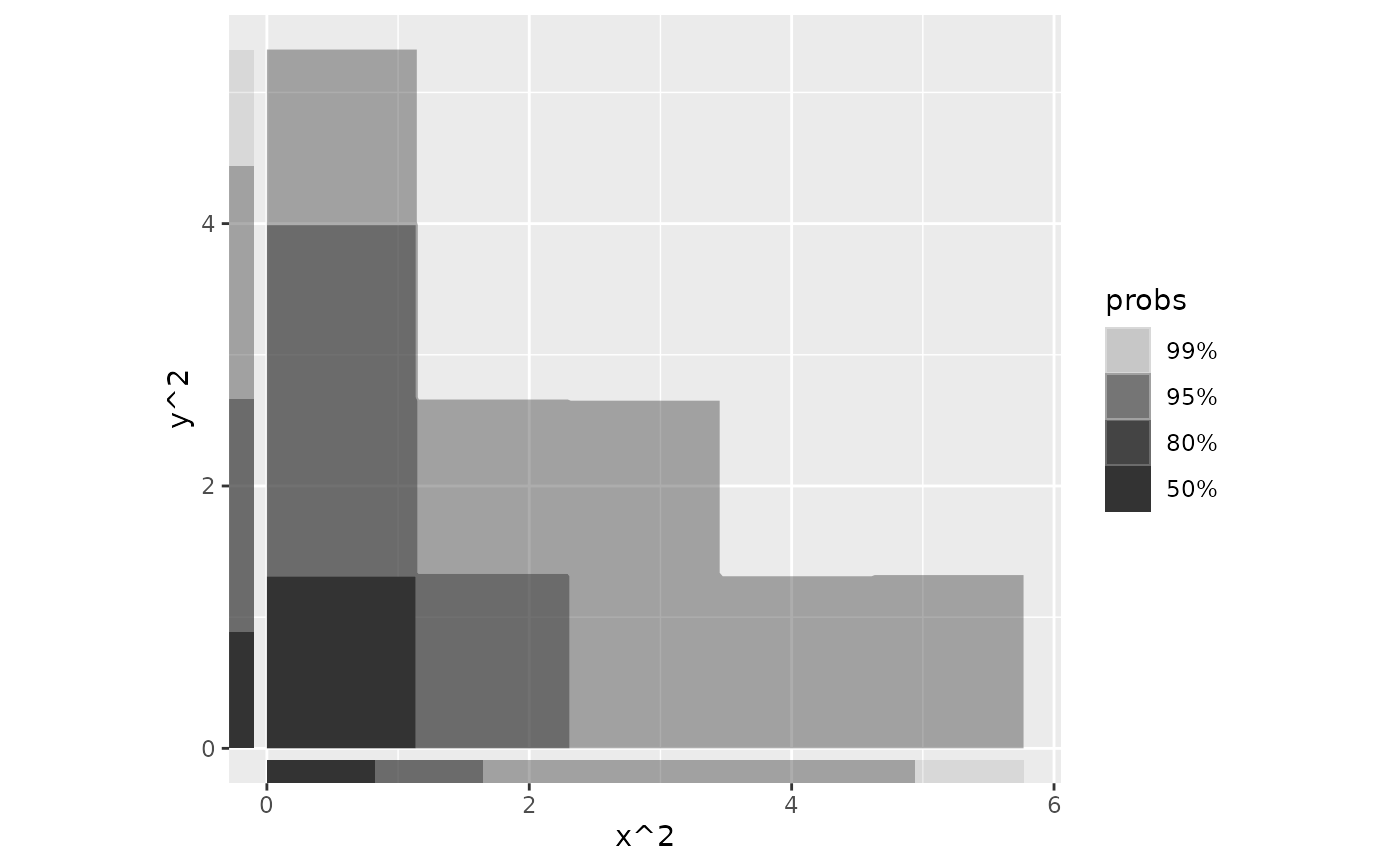Perform 1D density estimation, compute and plot the resulting highest density regions in a way similar to ggplot2::geom_rug(). Note, the plotted objects have probabilities mapped to the alpha aesthetic by default.

## Usage

stat_hdr_rug(
mapping = NULL,
data = NULL,
geom = "hdr_rug",
position = "identity",
...,
method = "kde",
method_y = "kde",
probs = c(0.99, 0.95, 0.8, 0.5),
xlim = NULL,
ylim = NULL,
n = 512,
na.rm = FALSE,
show.legend = TRUE,
inherit.aes = TRUE
)

geom_hdr_rug(
mapping = NULL,
data = NULL,
stat = "hdr_rug",
position = "identity",
...,
outside = FALSE,
sides = "bl",
length = unit(0.03, "npc"),
na.rm = FALSE,
show.legend = TRUE,
inherit.aes = TRUE
)

## Arguments

mapping

Set of aesthetic mappings created by aes(). If specified and inherit.aes = TRUE (the default), it is combined with the default mapping at the top level of the plot. You must supply mapping if there is no plot mapping.

data

The data to be displayed in this layer. There are three options:

If NULL, the default, the data is inherited from the plot data as specified in the call to ggplot().

A data.frame, or other object, will override the plot data. All objects will be fortified to produce a data frame. See fortify() for which variables will be created.

A function will be called with a single argument, the plot data. The return value must be a data.frame, and will be used as the layer data. A function can be created from a formula (e.g. ~ head(.x, 10)).

geom

The geometric object to use to display the data, either as a ggproto Geom subclass or as a string naming the geom stripped of the geom_ prefix (e.g. "point" rather than "geom_point")

position

Position adjustment, either as a string naming the adjustment (e.g. "jitter" to use position_jitter), or the result of a call to a position adjustment function. Use the latter if you need to change the settings of the adjustment.

...

Other arguments passed on to layer(). These are often aesthetics, used to set an aesthetic to a fixed value, like colour = "red" or size = 3. They may also be parameters to the paired geom/stat.

method, method_y

Density estimator(s) to use. By default method is used for both x- and y-axis. If specified, method_y will be used for y-axis. Accepts character vector: "kde","histogram", "freqpoly", or "norm". Alternatively accepts functions which return closures corresponding to density estimates, see ?get_hdr_1d or vignette("method", "ggdensity").

probs

Probabilities to compute highest density regions for.

xlim, ylim

Range to compute and draw regions. If NULL, defaults to range of data.

n

Resolution of grid defined by xlim and ylim. Ignored if method = "histogram" or method = "freqpoly".

na.rm

If FALSE, the default, missing values are removed with a warning. If TRUE, missing values are silently removed.

show.legend

logical. Should this layer be included in the legends? NA, the default, includes if any aesthetics are mapped. FALSE never includes, and TRUE always includes. It can also be a named logical vector to finely select the aesthetics to display.

inherit.aes

If FALSE, overrides the default aesthetics, rather than combining with them. This is most useful for helper functions that define both data and aesthetics and shouldn't inherit behaviour from the default plot specification, e.g. borders().

stat

The statistical transformation to use on the data for this layer, either as a ggproto Geom subclass or as a string naming the stat stripped of the stat_ prefix (e.g. "count" rather than "stat_count")

outside

logical that controls whether to move the rug tassels outside of the plot area. Default is off (FALSE). You will also need to use coord_cartesian(clip = "off"). When set to TRUE, also consider changing the sides argument to "tr". See examples.

sides

A string that controls which sides of the plot the rugs appear on. It can be set to a string containing any of "trbl", for top, right, bottom, and left.

length

A grid::unit() object that sets the length of the rug lines. Use scale expansion to avoid overplotting of data.

## Aesthetics

geom_hdr_rug understands the following aesthetics (required aesthetics are in bold):

• x

• y

• alpha

• fill

• group

• subgroup

## Computed variables

probs

The probability of the highest density region, specified by probs, corresponding to each point.

## Examples

set.seed(1)
df <- data.frame(x = rnorm(100), y = rnorm(100))

# Plot marginal HDRs for bivariate data
ggplot(df, aes(x, y)) +
geom_point() +
geom_hdr_rug() +
coord_fixed()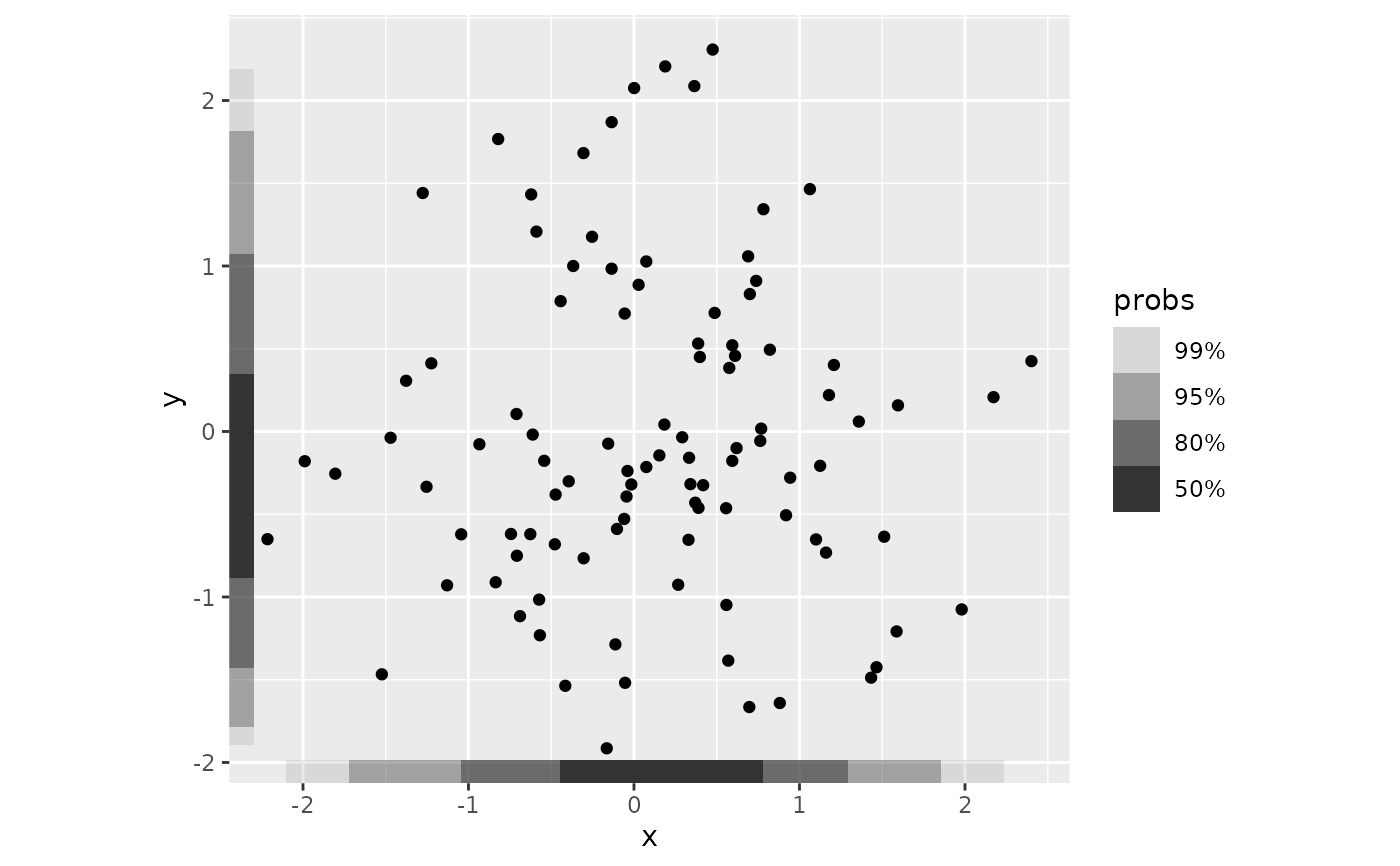ggplot(df, aes(x, y)) +
geom_hdr() +
geom_hdr_rug() +
coord_fixed()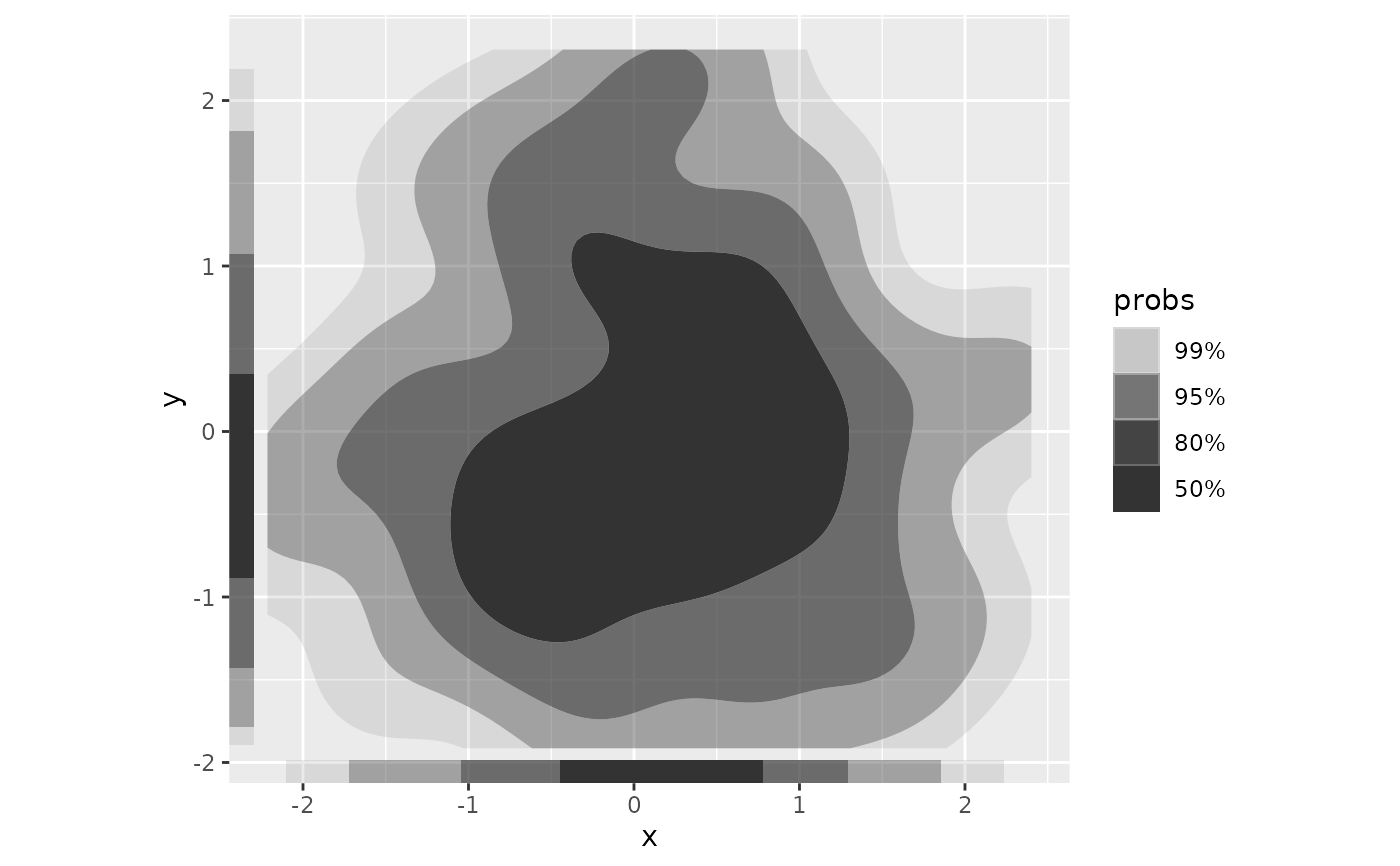# Plot HDR for univariate data
ggplot(df, aes(x)) +
geom_density() +
geom_hdr_rug()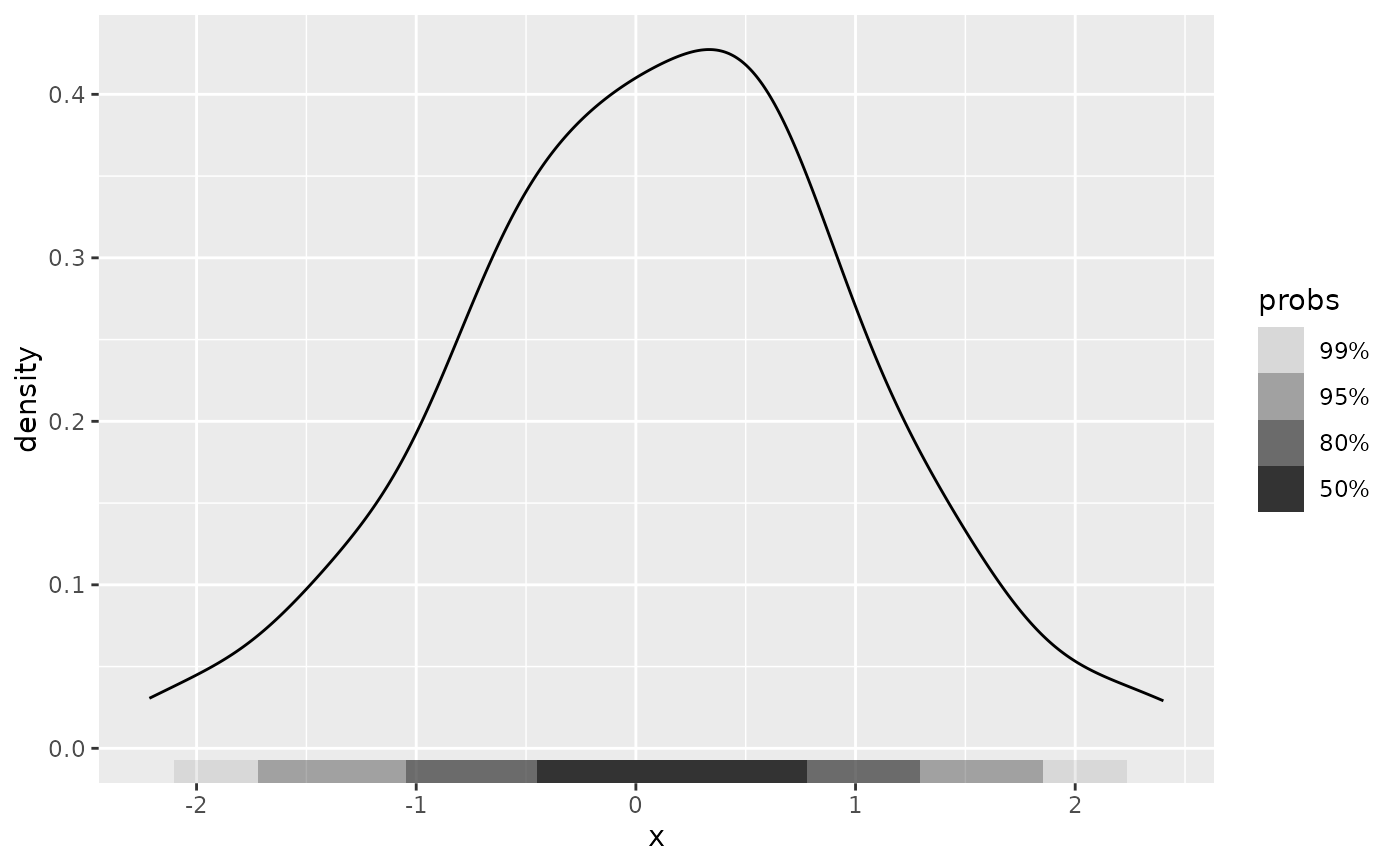ggplot(df, aes(y = y)) +
geom_density() +
geom_hdr_rug()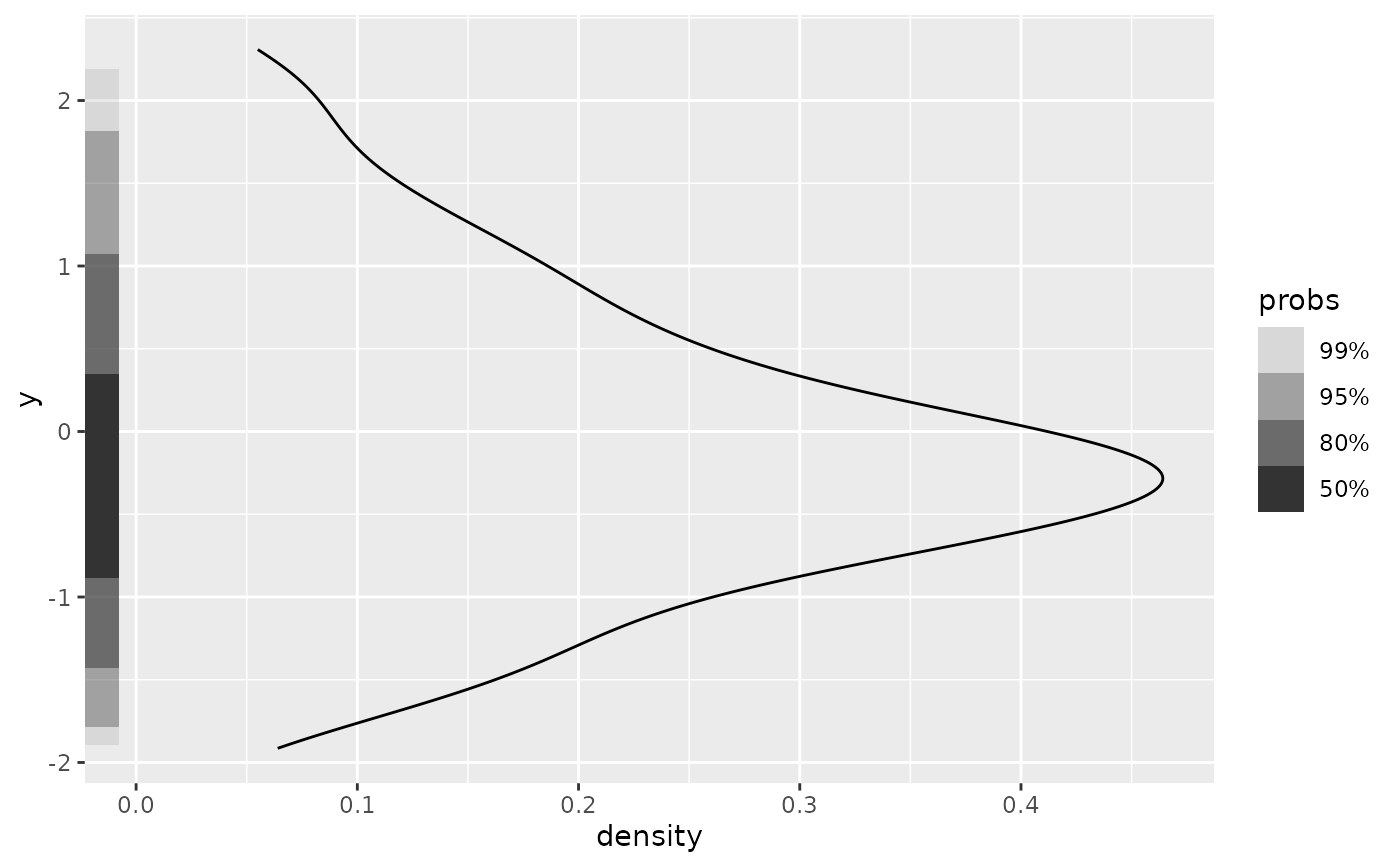# Specify location of marginal HDRs as in ggplot2::geom_rug()
ggplot(df, aes(x, y)) +
geom_hdr() +
geom_hdr_rug(sides = "tr", outside = TRUE) +
coord_fixed(clip = "off")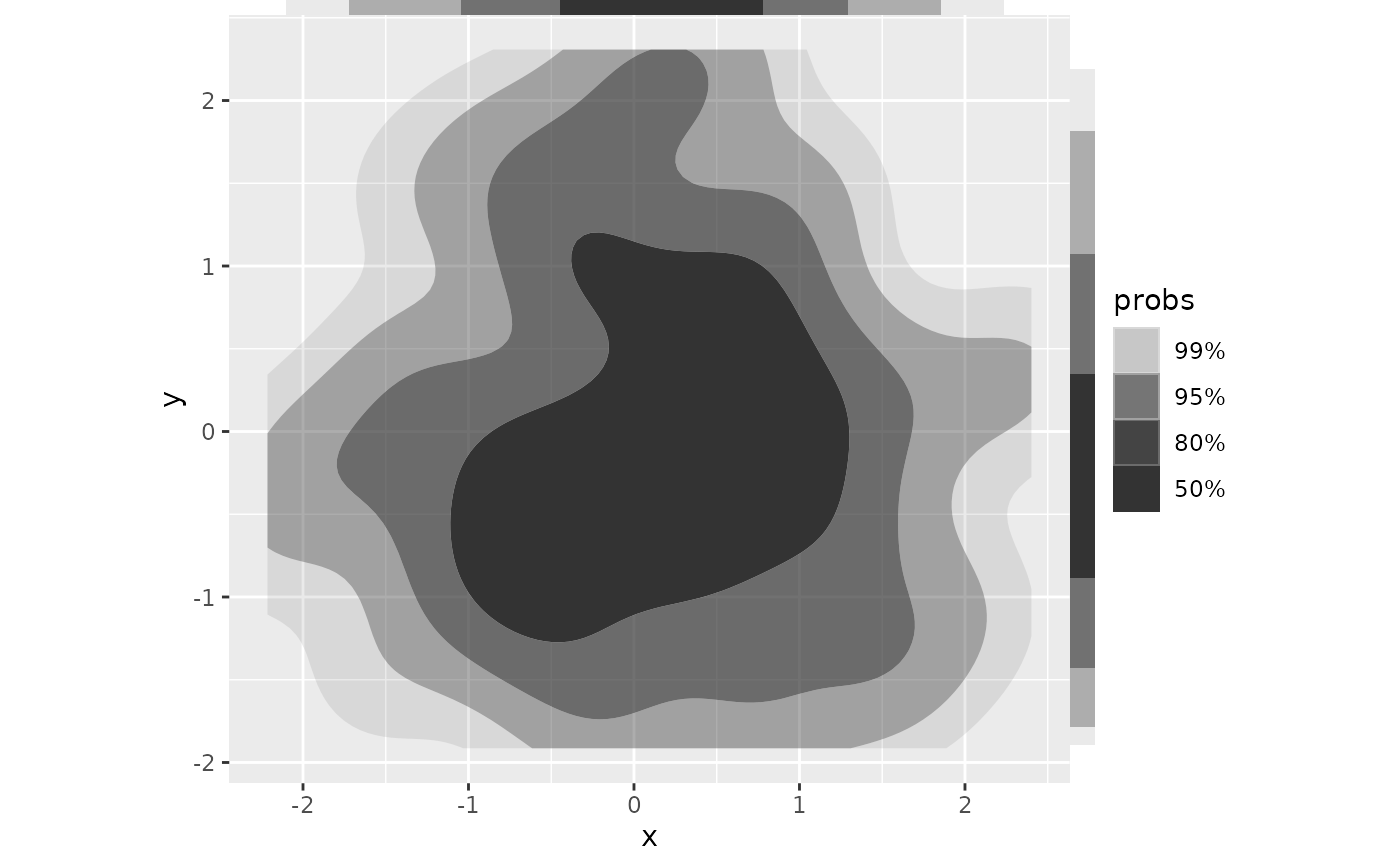# Can use same methods of density estimation as geom_hdr().
# For data with constrained support, we suggest setting method = "histogram":
ggplot(df, aes(x^2)) +
geom_histogram(bins = 30, boundary = 0) +
geom_hdr_rug(method = "histogram")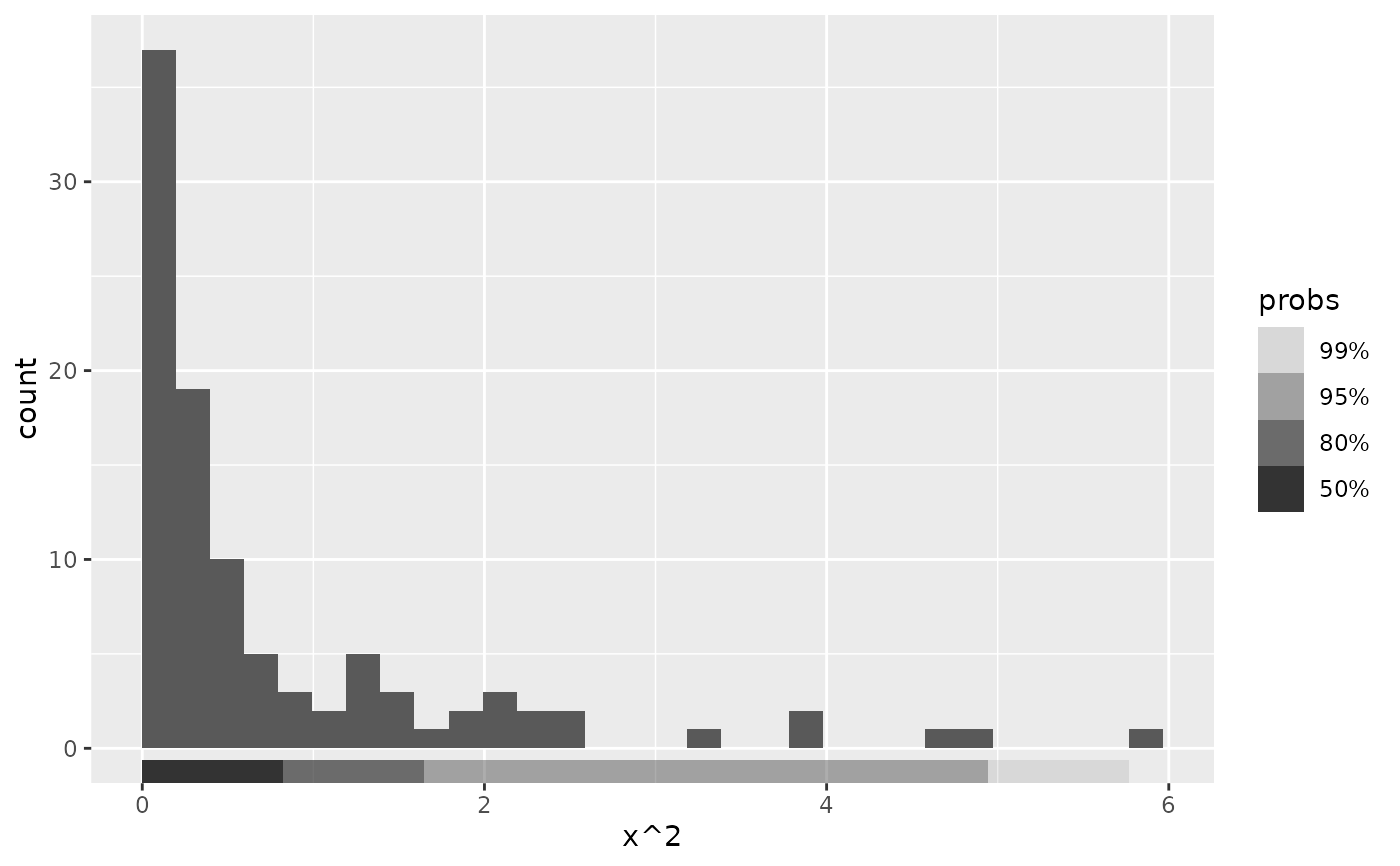ggplot(df, aes(x^2, y^2)) +
geom_hdr(method = "histogram") +
geom_hdr_rug(method = "histogram") +
coord_fixed()